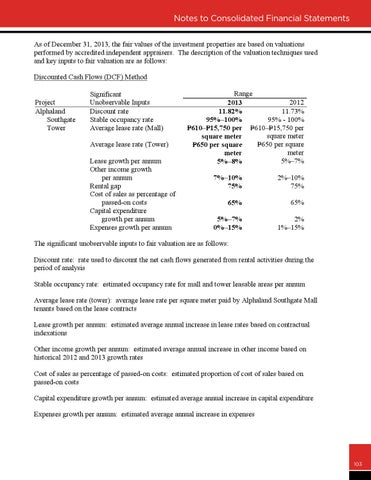to Consolidated Financial Statements - 58Notes As of December 31, 2013, the fair values of the investment properties are based on valuations performed by accredited independent appraisers. The description of the valuation techniques used and key inputs to fair valuation are as follows: Discounted Cash Flows (DCF) Method Project Alphaland Southgate Tower

Significant Unobservable Inputs Discount rate Stable occupancy rate Average lease rate (Mall) Average lease rate (Tower) Lease growth per annum Other income growth per annum Rental gap Cost of sales as percentage of passed-on costs Capital expenditure growth per annum Expenses growth per annum

Range 2012 2013 11.73% 11.82% 95% - 100% 95%–100% =610–P =15,750 per P =610–P =15,750 per P square meter square meter =650 per square P P =650 per square meter meter 5%–7% 5%–8% 7%–10% 75%

2%–10% 75%

65%

65%

5%–7% 0%–15%

2% 1%–15%

The significant unobservable inputs to fair valuation are as follows: Discount rate: rate used to discount the net cash flows generated from rental activities during the period of analysis Stable occupancy rate: estimated occupancy rate for mall and tower leasable areas per annum Average lease rate (tower): average lease rate per square meter paid by Alphaland Southgate Mall tenants based on the lease contracts Lease growth per annum: estimated average annual increase in lease rates based on contractual indexations Other income growth per annum: estimated average annual increase in other income based on historical 2012 and 2013 growth rates Cost of sales as percentage of passed-on costs: estimated proportion of cost of sales based on passed-on costs Capital expenditure growth per annum: estimated average annual increase in capital expenditure Expenses growth per annum: estimated average annual increase in expenses

103